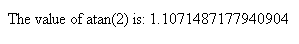Tutorials

JavaScript method atan()

This section illustrates you the use of JavaScript method atan(). The atan() method returns the arc tangent of a specified number as a numeric value between -PI/2 and PI/2 radians.

This section illustrates you the use of JavaScript method atan(). The atan() method returns the arc tangent of a specified number as a numeric value between -PI/2 and PI/2 radians.

JavaScript method atan()

This section illustrates you the use of JavaScript method atan(). The atan() method returns the arc tangent of a specified number as a numeric value between -PI/2 and PI/2 radians. You can see in the given example that we have used the method atan() and want  to find out the arc tangent of 2. We have created a button which calls the function calculate() from the script and displays the output.

Here is the code:



Use of atan() method

Output will be displayed as:On clicking the button, you will get the arc tangent of 2: